International
Tables for
Crystallography
Volume B
Reciprocal space
Edited by U. Shmueli

International Tables for Crystallography (2006). Vol. B. ch. 2.5, p. 287   | 1 | 2 |

## Section 2.5.3.2.2. Reciprocity and Friedel's law

P. Goodmanb

#### 2.5.3.2.2. Reciprocity and Friedel's law

| top | pdf |

Reciprocity was introduced into the subject of electron diffraction in stages, the essential theoretical basis, through Schrödinger's equation, being given by Bilhorn et al. (1964), and the N-beam diffraction applications being derived successively by von Laue (1935), Cowley (1969), Pogany & Turner (1968), Moodie (1972), Buxton et al. (1976), and Gunning & Goodman (1992).

Reciprocity represents a reverse-incidence configuration reached with the reversed wavevectors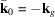and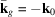, so that the scattering vector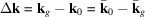is unchanged, but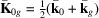is changed in sign and hence reversed (Moodie, 1972). The reciprocity equation,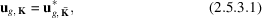is valid independently of crystal symmetry, but cannot contribute symmetry to the pattern unless a crystal-inverting symmetry element is present (since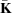belongs to a reversed wavevector). The simplest case is centrosymmetry, which permits the right-hand side of (2.5.3.1)to be complex-conjugated giving the useful CBED pattern equation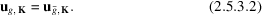Since K is common to both sides there is a point-by-point identity between the related distributions, separated by 2g (the distance between g andreflections). This invites an obvious analogy with Friedel's law,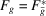, with the reservation that (2.5.3.2)holds only for centrosymmetric crystals. This condition (2.5.3.2)constitutes what has become known as the ±H symmetry and, incidentally, is the only reciprocity-induced symmetry so general as to not depend upon a disc symmetry-point or line, nor on a particular zone axis (i.e. it is not a point symmetry but a translational symmetry of the pattern intensity).

### ReferencesBilhorn, D. E., Foldy, L. L., Thaler, R. M. & Tobacman, W. (1964). Remarks concerning reciprocity in quantum mechanics. J. Math. Phys. 5, 435–441.Google ScholarBuxton, B., Eades, J. A., Steeds, J. W. & Rackham, G. M. (1976). The symmetry of electron diffraction zone axis patterns. Philos. Trans. R. Soc. London Ser. A, 181, 171–193.Google ScholarCowley, J. M. (1969). Image contrast in transmission scanning electron microscopy. Appl. Phys. Lett. 15, 58–59.Google ScholarGunning, J. & Goodman, P. (1992). Reciprocity in electron diffraction. Acta Cryst. A48, 591–595.Google ScholarLaue, M. von (1935). Die Fluoreszenzrontgenstrahlung von Einkristallen. Ann. Phys. (Leipzig), 23, 703–726.Google ScholarMoodie, A. F. (1972). Reciprocity and shape function in multiple scattering diagrams. Z. Naturforsch. Teil A, 27, 437–440.Google ScholarPogany, A. P. & Turner, P. S. (1968). Reciprocity in electron diffraction and microscopy. Acta Cryst. A24, 103–109.Google Scholar